# Cork and swimming

If a person weighs 80 kg, how many kilograms of cork must take a swimming belt to float on water? The density of the human body is 1050kg/m3 and cork 300kg/m3.

(Instructions: Let the human body and cork on a mixture with a density of 1000kg/m3. When you make the equation, you will compare the volumes of both components and the resulting mixture volume.)

x =  1.6327 kg

### Step-by-step explanation:

80/1050+x/300=(80+x)/1000

49x = 80

x = 80/49 ≈ 1.632653

Our simple equation calculator calculates it.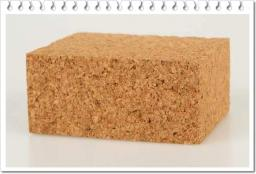Did you find an error or inaccuracy? Feel free to write us. Thank you!Tips to related online calculators
Do you have a system of equations and looking for calculator system of linear equations?
Tip: Our volume units converter will help you with the conversion of volume units.
Tip: Our Density units converter will help you with the conversion of density units.

## Related math problems and questions:

• MixingIf we mix 5 kg of goods of one kind and 3 kg second one, resulting mixture cost 16.50 EUR/kg. If these quantities are mixed in reverse - first three kilograms and 5 kilograms second cost of mixture is 18.50 EUR/kg. What is the price of one kg of goods of
• Reservoir + waterThe reservoir filled with water weighs 12 kg and after pouring off three-quarters of the water weights 3 kg. Calculate the weight and volume of the reservoir.
• Dried fruit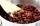The manufacturer produces a mixture of dried fruit. He purchased: 10kg pineapple for 200 Kc/kg 2kg papaya for 180 kc/kg 1kg of banana for 400 Kc/kg How many kgs of raisin for 80 Kc/kg must be put into the mix by the manufacturer so that the production pri
• Mixture of coffee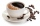They have to prepare a mixture of coffee in packing centres so that 1 kg costs 240 CZK. There are two types of coffee in stock at the price of 220 CZK per 1 kg and 300 CZK per 1 kg. How many kilograms of each species need to be mixed to prepare 50 kg of t
• Coffee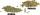Coffee merchant has coffee robusta and arabica species. 1 kg Robusta worth 450 CZK, Arabica 1 kg is 300 CZK more expensive. Calculate how many kilograms of Robusta and Arabica will need to produce 30 kg of the mixture so that the mixture cost is 490 CZK p
• Tea mixture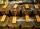Of the two sort of tea at a price of 180 CZK/kg and 240 CZK/kg we make a mixture 12 kg that should be prepared at a price of 200 CZK / kg. How many kilos of each sort of tea will we need to be mixed?
• Acid evaporationHow many kilograms of water do we have to evaporate from 100 kg of 32% acid to make it 80% concentration?
• Candies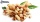In the confectionery, the price for 1 kg of pistachio candies cost CZK 360, and the price for 1 kg of hazelnut candies was CZK 280. Mixing these two types of sweets created a box of chocolates. How many grams of pistachios and how many grams of hazelnut c
• CoffeeOne kilogram of cheaper coffee costs CZK 150, one kilogram of more expensive coffee costs CZK 200. We have to prepare a mixture of 35 kg of coffee for 180 CZK. How do we prepare the mixture (how many of which kind of coffee do we need)?
• Floating of wood - Archimedes lawWhat will be the volume of the floating part of a wooden (balsa) block with a density of 200 kg/m3 and a volume of 0.02 m3 that floats in alcohol? (alcohol density is 789 kg/m3)
• Sea waterMixing 62 kg of sea water with 84 kg rainwater is created water containing 3.1% salt. How many percent sea water contains salt?
• RingThe ring made from gold and copper alloy has a weight of 14.5 g and a volume of 1.03 cm3. How much gold and how much copper it contains? The metal densities are Au 19.3 g/cm³ and Cu 8.94 kg·dm-3
• Float boya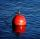A 0.5 meter spherical float is used as a location mark for a fishing boat anchor. It floats in salt water. Find the depth to which the float sinks if the material of which the float is made weighs 8 kilograms per cubic meter and salt water weighs 1027 kg/
• The aluminumThe aluminum object with a weight of 0.80 kg and a temperature of 250°C was placed in the water with a weight of 1.6 kg and a temperature of 23°C. What is the system temperature after reaching equilibrium? We assume that the heat exchange occurred only be
• Two teas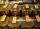A mixture weighing 10 kg was made from two types of tea. The price of tea was 160 CZK/kg and second tea 170 CZK/kg. The price of the mixture is 166 CZK/kg. How many kilograms of each type of tea had to be mixed?
• Water mixingWe have 520 ml of hot water and 640 ml of water at 48°C. What is the temperature of approximately hot water when the resulting mixture has a temperature of 65°C?
• SpiritFrom 55% and 80% spirit we would like to produce 0.2 kg of 60% spirit. How many of them we must use in a solution?